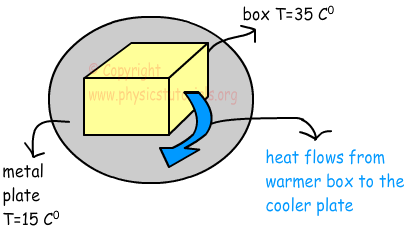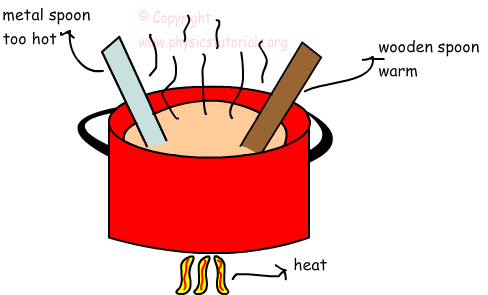Heat and Heat vs Temperature

HeatHeat is a form of energy that flows from hotter substance to colder one. We mean by hotter and colder substance, substance having high temperature and low temperature with respect to a reference matter. There must be a difference in temperatures of the substance to have heat or energy transfer. Heat is related to the quantity of matter also. If the object has big mass it also has big thermal energy and consequently amount of transferred energy increases. Since it is a type of energy we use Joule or Calories as unit of heat.Differences between Heat and Temperature

In daily life most of us use these terms interchangeably. In this section we learn differences between them.

• Heat is a type of energy, but temperature is not energy.
• Heat depends on mass of the substance, however; temperature does not depend on the quantity of matter. For example, temperature of one glass of boiling water and one teapot of boiling water are equal to each other; on the contrary they have different heat since they have different masses.
• You can measure temperature directly with a device called thermometer but heat cannot be measured with a device directly. You should know the mass, temperature and specific heat capacity of that matter.
• If you give heat to a matter, you increase its temperature or change its phase.
• Specific Heat Capacity

If you give same amount of heat to different type of matters you observe that changes in their temperatures are different. For instance, allyou experience that given an equal amount of heat to metal spoons and wooden spoons, metal spoon has greater change in its temperature. Thus, most of the housewives use wooden or plastic spoons while cooking. These examples show that each matter has its own characteristics to absorb heat. We call this concept as specific heat capacity of the matters. It is the distinguishing property of matters. We show it with the letter “c” and give the definition of it as, heat required to increase temperature of unit mass 1 ºC.On the contrary, heat capacity of the system is defined as “heat required increasing the temperature of whole substance” and we show it with “C”.

C=m.c where m is the mass of the substance and c is the specific heat of the matter.

With the help of specific heat capacity and mass of the matter we can find the relation between heat and change in the temperature in the given formula below.

Q=m.c.∆T

Where; Q is heat, m is mass, c is specific heat capacity and ∆T is the change in the temperature.

Heat Temperature and Thermal Expansion Exams and Solutions

Author: